# NCERT Solutions class-11 Maths Exercise 15.1## myCBSEguide App

CBSE, NCERT, JEE Main, NEET-UG, NDA, Exam Papers, Question Bank, NCERT Solutions, Exemplars, Revision Notes, Free Videos, MCQ Tests & more.

Exercise 15.1

Find the mean deviation about the mean for the data in Exercises 1 and 2.

1. 4, 7, 8, 9, 10, 12, 13, 17

Ans. Mean of the given data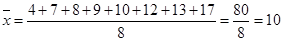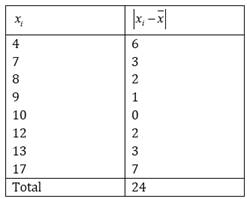Mean deviation about mean =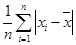=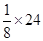= 3

2. 38, 70, 48, 40, 42, 55, 63, 46, 54, 44

Ans. Mean of the given data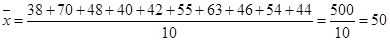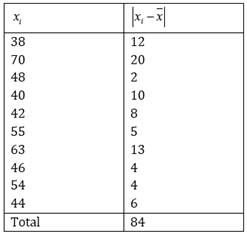Mean deviation about mean ==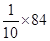= 8.4

Find the mean deviation about the median for the data in Exercises 3 and 4.

3. 13, 17, 16, 14, 11, 13, 10, 16, 11, 18, 12, 17

Ans. Arranging the data in ascending order, 10, 11, 12, 13, 13, 14, 16, 11, 18, 12, 17

Here,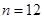(even number)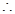Mean =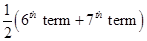=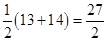= 13.5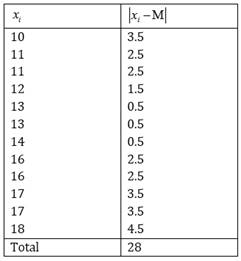Mean deviation about median =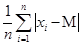=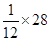= 2.33

4. 36, 72, 46, 42, 60, 45, 53, 46, 51, 49

Ans. Arranging the data in ascending order, 36, 42, 45, 46, 46, 49, 51, 53, 60, 72

Here,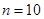(even number)Mean =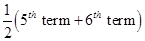=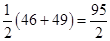= 47.5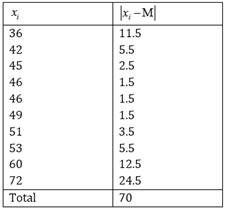Mean deviation about median ==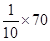= 7

Find the mean deviation about the mean for the data in Exercises 5 and 6.

5.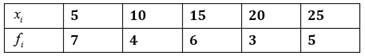Ans.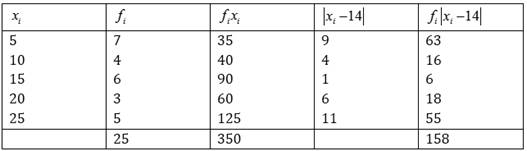Mean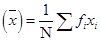=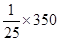= 14

Mean deviation about mean =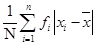=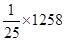= 6.32

6.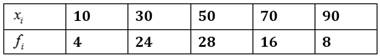Ans.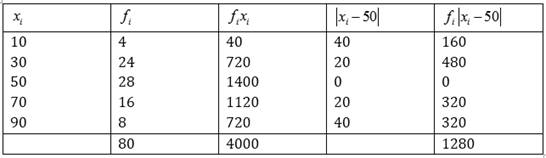Mean=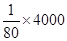= 50

Mean deviation about mean ==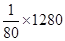= 16

Find the mean deviation about the median for the data in Exercises 7 and 8.

7.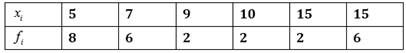Ans.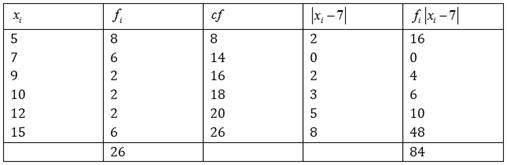Here,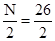= 13 and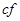= 7Median = 7

Mean deviation about median =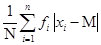=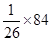= 3.23

8.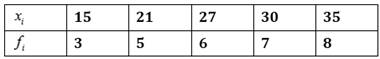Ans.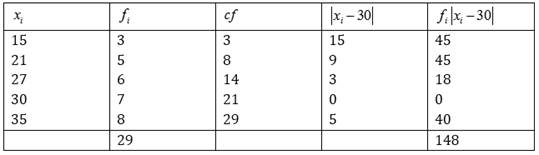Here,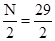= 14.5 and= 21Median = 30

Mean deviation about median ==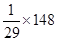= 5.1

Find the mean deviation about the mean for the data in Exercises 9 and 10.

9.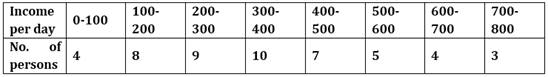Ans.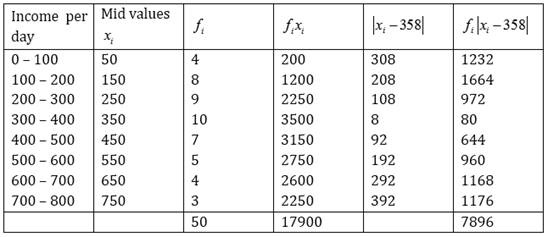Mean=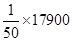= 358

Mean deviation about mean ==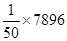= 157.92

10.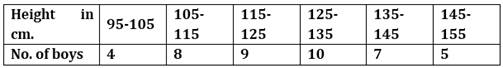Ans.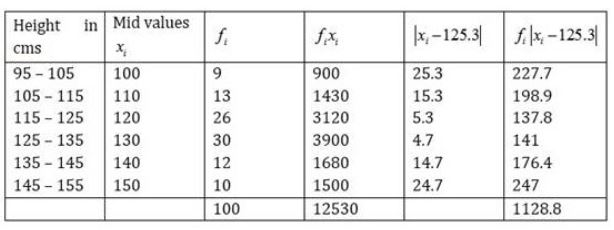Mean=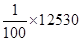= 125.3

Mean deviation about mean ==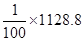= 11.28

11. Find the mean deviation about median for the following data: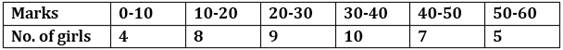Ans.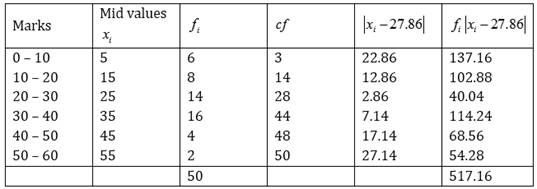Here,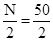= 25Median class = 20 – 30Median =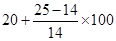= 20 + 7.86 = 27.86

Mean deviation about median ==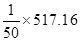= 10.37

12. Calculate the mean deviation about median age for the age distribution of 100 persons given below: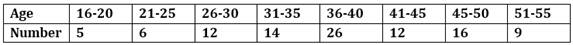Ans.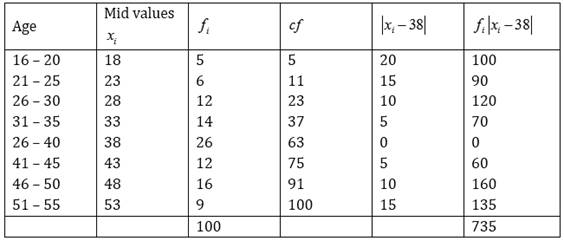Here,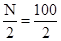= 50Median class = 35.5 – 40.5Median =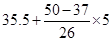= 35.5 + 2.5 = 38

Mean deviation about median ==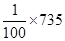= 7.35### 6 thoughts on “NCERT Solutions class-11 Maths Exercise 15.1”

1. Bhut hi achi site ha smjh ma assani sa aagya

2. Error: for Q.5) mean deviation about mean=158/25=6.32 not 1258/25

3. Bilkul bekar sara short cut me likha hua hai puri shi se smja nhi rhe ho??

4. Thanks

5. Boht Achha a simple way se btaya hai

6. Samjane ka trika bda acha h bilkul hamare sir ki tarah ???